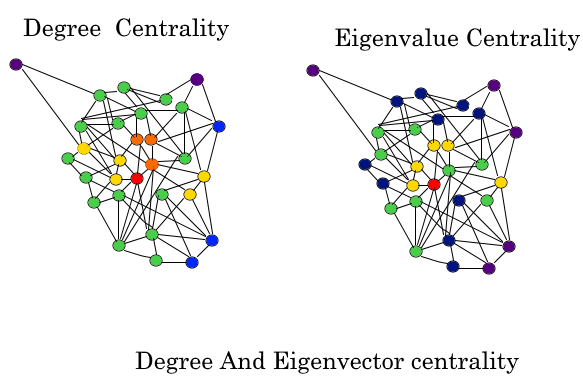# Eigenvector Centrality (Centrality Measure)

In graph theory, eigenvector centrality (also called eigencentrality) is a measure of the influence of a node in a network. It assigns relative scores to all nodes in the network based on the concept that connections to high-scoring nodes contribute more to the score of the node in question than equal connections to low-scoring nodes.

Google’s PageRank and the Katz centrality are variants of the eigenvector centrality.

Using the adjacency matrix to find eigenvector centrality

For a given graphwithvertices letbe the adjacency matrix, i.e.if vertexis linked to vertex, andotherwise. The relative centrality score of vertexcan be defined as:whereis a set of the neighbors ofandis a constant. With a small rearrangement this can be rewritten in vector notation as the eigenvector equationIn general, there will be many different eigenvaluesfor which a non-zero eigenvector solution exists. However, the additional requirement that all the entries in the eigenvector be non-negative implies (by the Perron–Frobenius theorem) that only the greatest eigenvalue results in the desired centrality measure. Thecomponent of the related eigenvector then gives the relative centrality score of the vertexin the network. The eigenvector is only defined up to a common factor, so only the ratios of the centralities of the vertices are well defined. To define an absolute score one must normalise the eigen vector e.g. such that the sum over all vertices is 1 or the total number of vertices n. Power iteration is one of many eigenvalue algorithms that may be used to find this dominant eigenvector. Furthermore, this can be generalized so that the entries in A can be real numbers representing connection strengths, as in a stochastic matrix.Following is the code for the calculation of the Eigen Vector Centrality of the graph and its various nodes.

 def eigenvector_centrality(G, max_iter=100, tol=1.0e-6, nstart=None,                             weight='weight'):      """Compute the eigenvector centrality for the graph G.         Eigenvector centrality computes the centrality for a node based on the      centrality of its neighbors. The eigenvector centrality for node i is         .. math::             \mathbf{Ax} = \lambda \mathbf{x}         where A is the adjacency matrix of the graph G with eigenvalue \lambda.      By virtue of the Perron–Frobenius theorem, there is a unique and positive      solution if \lambda is the largest eigenvalue associated with the      eigenvector of the adjacency matrix A (_).         Parameters      ----------      G : graph        A networkx graph         max_iter : integer, optional        Maximum number of iterations in power method.         tol : float, optional        Error tolerance used to check convergence in power method iteration.         nstart : dictionary, optional        Starting value of eigenvector iteration for each node.         weight : None or string, optional        If None, all edge weights are considered equal.        Otherwise holds the name of the edge attribute used as weight.         Returns      -------      nodes : dictionary         Dictionary of nodes with eigenvector centrality as the value.                  Notes      ------      The eigenvector calculation is done by the power iteration method and has      no guarantee of convergence. The iteration will stop after max_iter      iterations or an error tolerance of number_of_nodes(G)*tol has been      reached.         For directed graphs this is "left" eigenvector centrality which corresponds      to the in-edges in the graph. For out-edges eigenvector centrality      first reverse the graph with G.reverse().               """     from math import sqrt      if type(G) == nx.MultiGraph or type(G) == nx.MultiDiGraph:          raise nx.NetworkXException("Not defined for multigraphs.")         if len(G) == 0:          raise nx.NetworkXException("Empty graph.")         if nstart is None:             # choose starting vector with entries of 1/len(G)          x = dict([(n,1.0/len(G)) for n in G])      else:          x = nstart         # normalize starting vector      s = 1.0/sum(x.values())      for k in x:          x[k] *= s      nnodes = G.number_of_nodes()         # make up to max_iter iterations      for i in range(max_iter):          xlast = x          x = dict.fromkeys(xlast, 0)             # do the multiplication y^T = x^T A          for n in x:              for nbr in G[n]:                  x[nbr] += xlast[n] * G[n][nbr].get(weight, 1)             # normalize vector          try:              s = 1.0/sqrt(sum(v**2 for v in x.values()))             # this should never be zero?          except ZeroDivisionError:              s = 1.0         for n in x:              x[n] *= s             # check convergence          err = sum([abs(x[n]-xlast[n]) for n in x])          if err < nnodes*tol:              return x         raise nx.NetworkXError("""eigenvector_centrality():  power iteration failed to converge in %d iterations."%(i+1))""")

The above function is invoked using the networkx library and once the library is installed, you can eventually use it and the following code has to be written in python for the implementation of the eigen vector centrality of a node.

 >>> import networkx as nx  >>> G = nx.path_graph(4)  >>> centrality = nx.eigenvector_centrality(G)  >>> print(['%s %0.2f'%(node,centrality[node]) for node in centrality])

The output of the above code is:

 ['0 0.37', '1 0.60', '2 0.60', '3 0.37']

The above result is a dictionary depicting the value of eigen vector centrality of each node. The above is an extension of my article series on the centrality measures. Keep networking!!!

References

https://en.wikipedia.org/wiki/Eigenvector_centrality

My Personal Notes arrow_drop_upCheck out this Author's contributed articles.

If you like GeeksforGeeks and would like to contribute, you can also write an article using contribute.geeksforgeeks.org or mail your article to contribute@geeksforgeeks.org. See your article appearing on the GeeksforGeeks main page and help other Geeks.

Please Improve this article if you find anything incorrect by clicking on the "Improve Article" button below.

Article Tags :

Be the First to upvote.

Please write to us at contribute@geeksforgeeks.org to report any issue with the above content.